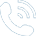01527 543361

Mathematics

 Autumn 1 Autumn 2 Spring 1 Spring 2 Summer 1 Summer 2 Year 5 Number and place value, approximation and estimation/Rounding. Calculations 1 Geometry- Properties of shapes 1. Calculations 2. Statistics Calculations 3. Fractions, decimals and percentages 1 Geometry- Position and direction. Measurement 1. Algebra 1. Geometry- Position and direction. Measurement 1. Algebra 1. Measurements 3. Consolidation and application. Year 6 Counting, partitioning and calculating- Unit A1. Securing number facts and understanding shape- Unit B1. Handling data and measures- Unit C1. Calculating, measuring and understanding shape - Unit D1. Securing number facts, relationships and calculating- Unit E1. Counting, partitioning and calculating- Unit A2. Securing number facts and understanding shape- Unit B2. Handling data and measures- Unit C2. Calculating, measuring and understanding shape- Unit D2. Securing number facts, relationships and calculating- Unit E2. Counting, partitioning and calculating- Unit A3. Securing number facts and understanding shape- Unit B3. Handling data and measures- Unit C3. Calculating, measuring and understanding shape- Unit D3. Securing number facts, relationships and calculating- Unit E3. Year 7 Algebra 1-sequences. Number 1- decimals Shape, space and measures 1- area and perimeter. Number 2-Fractions, decimals and percentages. Handling data 1-graphs, probability and averages. Algebra 2- equations and formulae. Shape, space and measures 2- angles Handling data 2-presenting data. Number 3- calculating.  Algebra 3- functions. Shape, space and measures 3- constructions. Number 4- ratio and proportion. Algebra 4- problem solving. Shape, space and measures 4- symmetry and transformations.  Handling data 3-pie charts and surveys. Number 5- decimals and calculating. Algebra 5- using algebra. Shape, space and measures 5-polygons. Year 8 Number and algebra 1- properties of numbers and sequences. Algebra 2- solving equations, simplifying and formulae. eometry and measures 1- Properties of shapes and angles.   Statistics 1- probability. Number 2- fractions, decimals and percentages.   Algebra 3- linear functions and graphs. Geometry and measures 2- area, volume and surface area.   Statistics 2- graphs Number 3- calculations and using a calculator.   Geometry and measures 3- ratio and transformations. Statistics 3- data collection and interpretation.   Geometry and measures 4- co-ordinates and constructions.   Solving problems.

Key Stage 2 SATs revision answers:

Ten for ten answer pack - Egg-ceeding

Ten for ten answer pack - Egg-spected

Ten for ten answer pack - Egg-stra support